# VBA Matrix – Create & More

This tutorial will show you how to  create a matrix using an Array in VBA.

A matrix is a rectangular array of numbers with the same amount of Rows and Columns. You can create a matrix in VBA by populating Array variables and using VBA Loops.

## Creating a Matrix in VBA

``````Sub CreateSimpleMatrix()
Dim matrix() As Integer
Dim x, i, j, k As Integer
're-dim the size of the array
ReDim matrix(1 To 3, 1 To 3) As Integer
x = 1
For i = 1 To 3
For j = 1 To 3
matrix(i, j) = x
x = (x + 1)
Next j
Next i
' return result to sheet in one go
Range("A1:C3") = matrix
End Sub``````

Running the procedure above will return a simple matrix to Range(“A1:C3”) to your worksheet.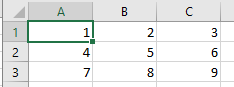## Convert Single Row Vector into a Matrix

Consider the column of numbers below. You may at some stage wish to convert a column of numbers to a matrix.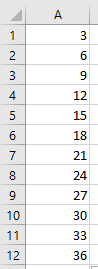Using the function below, we can create a matrix from the list of numbers.

``````Function Create_Matrix(Vector_Range As Range, No_Of_Cols_in_output As Integer, No_of_Rows_in_output As Integer) As Variant
ReDim Temp_Array(1 To No_Of_Cols_in_output, 1 To No_of_Rows_in_output)
Dim No_Of_Elements_In_Vector As Integer
Dim Col_Count As Integer, Row_Count As Integer

No_Of_Elements_In_Vector = Vector_Range.Rows.Count

'Eliminate NULL Conditions
If Vector_Range Is Nothing Then Exit Function
If No_Of_Cols_in_output = 0 Then Exit Function
If No_of_Rows_in_output = 0 Then Exit Function
If No_Of_Elements_In_Vector = 0 Then Exit Function

For Col_Count = 1 To No_Of_Cols_in_output
For Row_Count = 1 To No_of_Rows_in_output
Temp_Array(Col_Count, Row_Count) = Vector_Range.Cells(((No_of_Rows_in_output) * (Col_Count - 1) + Row_Count), 1)
Next Row_Count
Next Col_Count
Create_Matrix = Temp_Array

End Function``````

We can create the matrix in our Excel sheet, by calling the function above.

``````Sub ConvertToMatrix()
Range("C1:H2") = Create_Matrix(Range("A1:A10"), 2, 6)
End Sub``````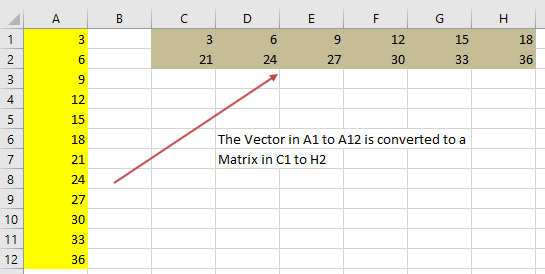## Convert a Matrix into a Single Row Vector

Alternatively, you may want to convert a Matrix into a Single row. Consider the Matrix below.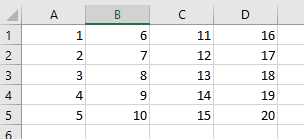Using the function below, we can convert this into a single dimension array.

``````Function Create_Vector(Matrix_Range As Range) As Variant
Dim No_of_Cols As Integer, No_Of_Rows As Integer
Dim i As Integer
Dim j As Integer
'pick up the rows and columns from the matrix
No_of_Cols = Matrix_Range.Columns.Count
No_Of_Rows = Matrix_Range.Rows.Count
ReDim Temp_Array(No_of_Cols * No_Of_Rows)
'Eliminate NULL Conditions
If Matrix_Range Is Nothing Then Exit Function
If No_of_Cols = 0 Then Exit Function
If No_Of_Rows = 0 Then Exit Function
'loop through the array - the first element
For j = 1 To No_Of_Rows
'now loop through the second element
For i = 0 To No_of_Cols - 1
'assign to a single dimension temporary array
Temp_Array((i * No_Of_Rows) + j) = Matrix_Range.Cells(j, i + 1)
Next i
Next j
Create_Vector = Temp_Array
End Function``````

We can now call this function using the procedure below.

``````Sub GenerateVector()
Dim Vector() As Variant
Dim k As Integer
Dim No_of_Elements
'get the array
Vector = Create_Vector(Sheets("Sheet1").Range("A1:D5"))
'loop through the array and populate the sheet
For k = 0 To UBound(Vector) - 1
Sheets("Sheet1").Range("G1").Offset(k, 0).Value = Vector(k + 1)
Next k
End Sub``````

This procedure will return the following result.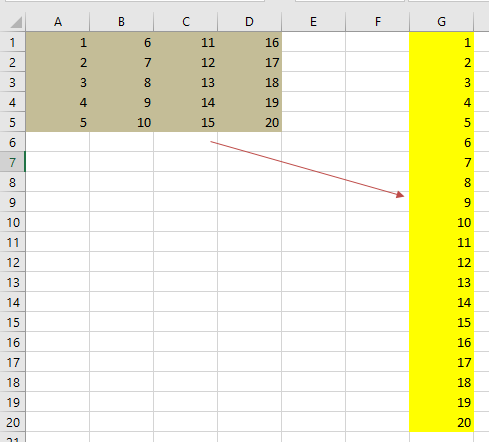## Using the WorksheetFunction.MMULT to create a Matrix Array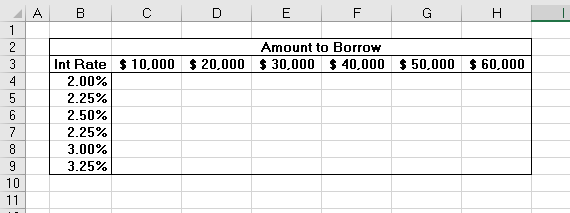We can use the procedure below to calculate the interest amount for the grid above based on the interest rate and the amount the person wants to borrow.

``````Sub UseMMULT()
Dim rngIntRate As Range
Dim rngAmtLoan As Range
Dim Result() As Variant
'populate our range objects
Set rngIntRate = Range("B4:B9")
Set rngAmtLoan = Range("C3:H3")
'use the MMULT formula to fill the result array
Result = WorksheetFunction.MMult(rngIntRate, rngAmtLoan)
'populate the sheet
Range("C4:H9") = Result
End Sub``````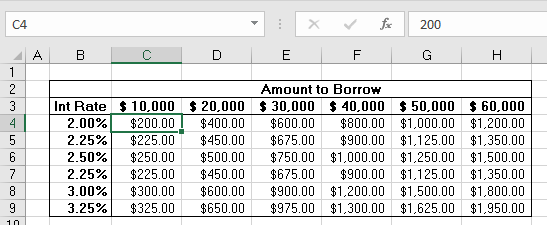You will notice in the sheet above, that procedure above populates the cells with values rather than formulas – see C4 in the above graphic – it has the value 200 in it, not a formula.  Using the WorksheetFunction method always returns a static value to the worksheet and not a formula.  This means that should the interest rate or loan amount change, the corresponding values in the filled in matrix WILL NOT change.

Instead of using the WorksheetFunction.MMULT, you can use VBA to apply the MMULT Function to a cell using the FormulaArray method.

``````Sub InsertMMULT()
Range("C4:H9").FormulaArray = "=MMULT(B4:B9,C3:H3)"
End Sub``````

Notice that now when the spreadsheet is populated, a formula is used in the cells.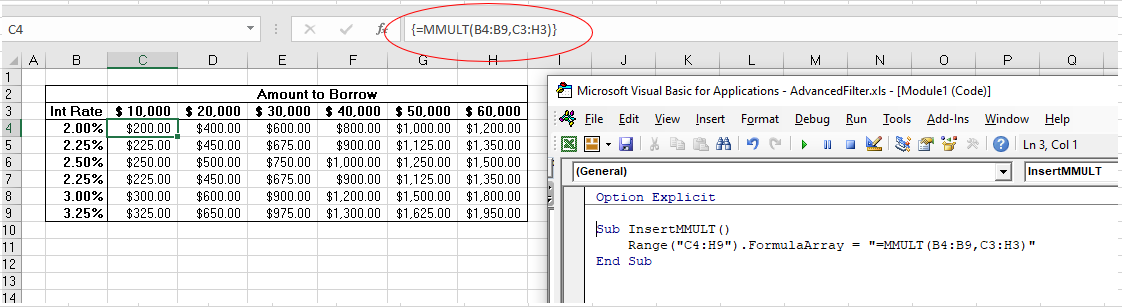Stop searching for VBA code online. Learn more about AutoMacro - A VBA Code Builder that allows beginners to code procedures from scratch with minimal coding knowledge and with many time-saving features for all users!